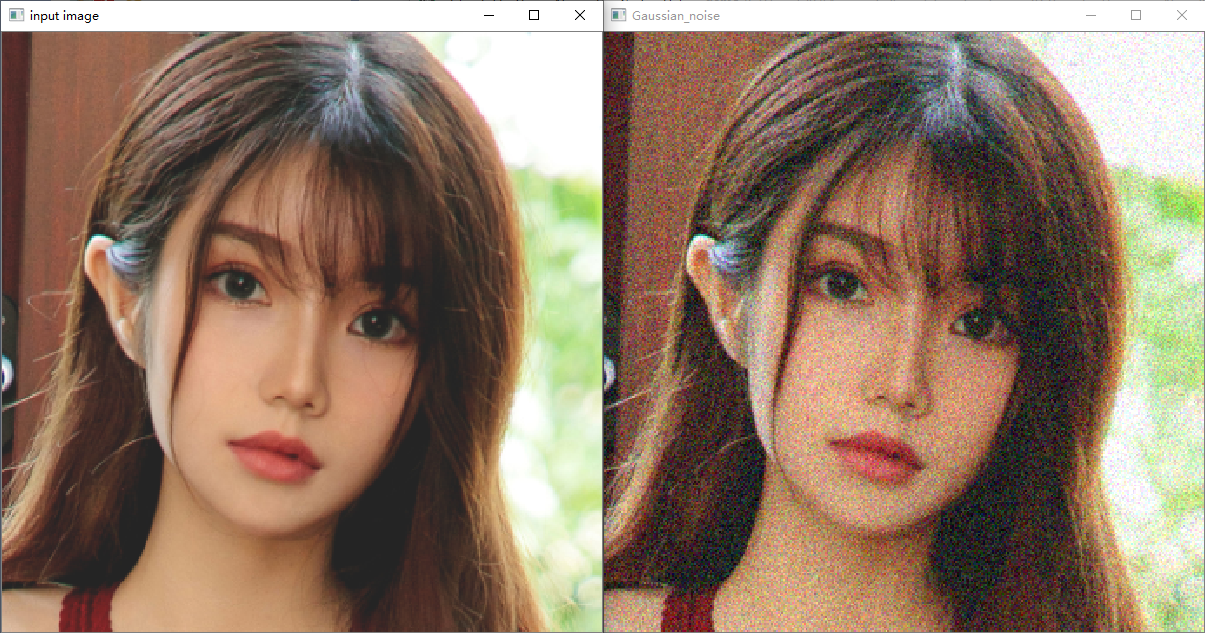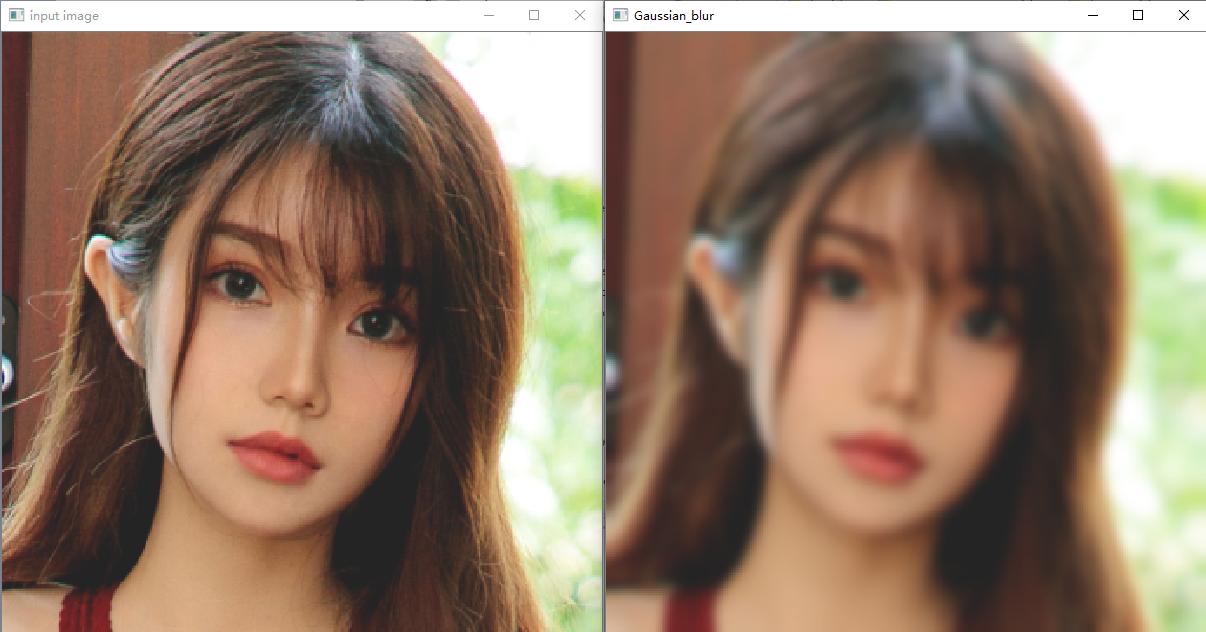# 图像处理中的高斯噪声

## 图像处理-图像添加高斯噪声并滤波

2015-03-27 21:06:09 u013045749 阅读数 6985
• ###### matlab图像专题技术案例精讲

建议按照课程顺序学习，帮助大家更好的掌握该领域的应用和知识，并在实战编程深入学习和开展研究。相信经过努力学习和反复实践，可以取得收获。

69课时 918分钟 364人学习 刘昱显
免费试看MATLAB代码如下：

```clear; clc; close all;

%% 添加高斯白噪声
im_gaus = imnoise(im, 'gaussian', 0, 0.02); % 添加均值为0，方差为0.02的高斯白噪声
figure('NumberTitle', 'off', 'Name', 'smooth apple with gaussian noise');
subplot(3, 4, 1);
imshow(im); % 显示原始图像
title('apple-Original');
subplot(3, 4, 2);
imshow(im_gaus); % 显示添加高斯白噪声的图像
title('apple-gaussian noise');

%% 图像滤波
im_gray = rgb2gray(im_gaus); % 彩色图转灰度图
im_bw = im2bw(im_gray, 0.9); %灰度图转二值图
subplot(3, 4, 3);
imshow(im_gray); % 显示灰度图
title('apple-gray');
subplot(3, 4, 4);
imshow(im_bw); % 显示二值图
title('apple-binary');
% 中值滤波
im_med = medfilt2(im_bw);
im_med = medfilt2(im_med);
im_med = medfilt2(im_med);
subplot(3, 4, 5);
imshow(im_med); % 显示中值滤波后的图像
title('apple-medfilt_3');
% 高斯滤波
h = fspecial('gaussian', [18 18], 0.15);
im_gausfilt = imfilter(im_bw, h);
subplot(3, 4, 6);
imshow(im_gausfilt); % 显示高斯低通滤波后的图像
title('apple-gausfilt');
im_gf_med = medfilt2(im_gausfilt); % 对高斯滤波后的图像进行中值滤波
subplot(3, 4, 7);
imshow(im_gf_med); % 显示高斯+中值滤波后的图像
title('apple-gaus-med');
% 均值滤波
h = fspecial('average', [5, 5]);
im_mean = imfilter(im_bw, h);
subplot(3, 4, 8);
imshow(im_mean); % 显示均值滤波后的图像
title('apple-mean');
% 形态学滤波
se = strel('disk', 2); %  创建一个平坦的圆盘型结构元素，半径为2
% 闭运算：先膨胀再腐蚀
im_clos = imclose(im_bw, se);
subplot(3, 4, 9);
imshow(im_clos);
title('imclose');
% 闭运算之后再进行中值滤波
im_clos_med = medfilt2(im_clos);
subplot(3, 4, 10);
imshow(im_clos_med);
title('imclose+med');
% 开运算：先腐蚀再膨胀
im_op = imopen(im_bw, se);
% im_op = imopen(im_clos_med, se); % 开运算进行孔洞填充
subplot(3, 4, 11);
imshow(im_op);
title('impen');
% 利用imfill对图像进行孔洞填充
im_clos_med_fil = imfill(im_clos_med);```

## 数字图像处理——添加高斯噪声&椒盐噪声

2016-11-17 15:38:54 u012936765 阅读数 46062
• ###### matlab图像专题技术案例精讲

建议按照课程顺序学习，帮助大家更好的掌握该领域的应用和知识，并在实战编程深入学习和开展研究。相信经过努力学习和反复实践，可以取得收获。

69课时 918分钟 364人学习 刘昱显
免费试看

# 1.添加高斯噪声

## 1.1 概率密度函数σ为z的标准差,z为均值,用E。

## 1.2 生成高斯分布随机数序列

``````double generateGaussianNoise(double mu, double sigma)
{
static double V1, V2, S;
static int phase = 0;
double X;
double U1,U2;
if ( phase == 0 ) {
do {
U1 = (double)rand() / RAND_MAX;
U2 = (double)rand() / RAND_MAX;

V1 = 2 * U1 - 1;
V2 = 2 * U2 - 1;
S = V1 * V1 + V2 * V2;
} while(S >= 1 || S == 0);

X = V1 * sqrt(-2 * log(S) / S);
} else{
X = V2 * sqrt(-2 * log(S) / S);
}
phase = 1 - phase;
return mu+sigma*X;
}``````

## 1.3 添加高斯噪声

``````void AddNoise(Mat img,double mu, double sigma,int k){
Mat outImage;
outImage.create(img.rows,img.cols,img.type());
for(int x=0;x<img.rows;x++){
for(int y=0;y<img.cols;y++){
double temp = img.at<uchar>(x, y)
+k*generateGaussianNoise(mu,sigma);
if(temp>PixcelMax)
temp=PixcelMax;
else if(temp<PixcelMin)
temp=PixcelMin;
outImage.at<uchar>(x, y) = temp;
}
}
Filter(outImage,Filter::NXBJZ,3,3,1);
imshow("Output", outImage);
cvWaitKey(0);
}
``````

## 1.4 效果图# 2.添加椒盐噪声

## 2.1 概率密度函数## 2.2 添加椒盐噪声

``````void AddNoise(Mat img,double SNR ){
Mat outImage;
outImage.create(img.rows,img.cols,img.type());
int SP = img.rows*img.cols;
int NP = SP*(1-SNR);
outImage = img.clone();
for(int i=0; i<NP; i++) {

int x = (int)(rand()*1.0/RAND_MAX* (double)img.rows);
int y = (int)(rand()*1.0/RAND_MAX* (double)img.cols);
int r = rand()%2;
if(r){
outImage.at<uchar>(x, y)=0;
}
else{
outImage.at<uchar>(x, y)=255;
}

}
Filter(outImage,Filter::NXBJZ,3,3,1);
imshow("Output", outImage);
cvWaitKey(0);
}``````

## 2.3 效果图# 3.均值滤波器

## 3.1 算术均值滤波器Sx,y为以(x,y)为中心,长为m宽为n的矩形范围。

### 3.1.1 滤除高斯噪声### 3.1.2滤除椒盐噪声## 3.2 几何均值滤波器Sx,y为以(x,y)为中心,长为m宽为n的矩形范围。

### 3.2.1 滤除高斯噪声### 3.2.2 滤除椒盐噪声## 3.3 谐波均值滤波器### 3.3.1 滤除高斯噪声### 3.3.2滤除椒盐噪声## 3.4 逆谐波均值滤波器### 3.4.1 滤除高斯噪声### 3.4.2滤除椒盐噪声Q为正时,会对灰度值较大的噪声进行放大,Q为负时,会对灰度值较小的噪声进行 放大。

``````void Filter(Mat img,Filter f,int m,int n,int Q = 0){
Mat outImage;
outImage.create(img.rows,img.cols,img.type());
int h = m/2,w=n/2;
for(int x=0;x<img.rows;x++){
for(int y=0;y<img.cols;y++){
int cnt = 0;
if(f== Filter::SSJZ){
long double sum = 0;
for(int i=x-h;i<=x+h;i++){
for(int j=y-w;j<=y+w;j++){
if(i<0||i>=img.rows||j<0||j>=img.cols)continue;
cnt++;
sum+=img.at<uchar>(i, j);
}
}
outImage.at<uchar>(x, y)=sum/cnt;
}
else if(f== Filter::JHJZ){
long double sum = 1;
for(int i=x-h;i<=x+h;i++){
for(int j=y-w;j<=y+w;j++){
if(i<0||i>=img.rows||j<0||j>=img.cols)continue;
cnt++;
sum*=img.at<uchar>(i, j);
}
}
outImage.at<uchar>(x, y)=pow(sum,1.0/cnt);
}
else if(f== Filter::XBJZ){
long double sum = 0;
for(int i=x-h;i<=x+h;i++){
for(int j=y-w;j<=y+w;j++){
if(i<0||i>=img.rows||j<0||j>=img.cols)continue;
cnt++;
sum+=1.0/img.at<uchar>(i, j);
}
}
outImage.at<uchar>(x, y)=cnt/sum;
}
else if(f== Filter::NXBJZ){
long double sum1 = 0,sum2 = 0;
for(int i=x-h;i<=x+h;i++){
for(int j=y-w;j<=y+w;j++){
if(i<0||i>=img.rows||j<0||j>=img.cols)continue;
cnt++;
sum1+=pow(img.at<uchar>(i, j),Q);
sum2+=pow(img.at<uchar>(i, j),Q+1);
}
}
outImage.at<uchar>(x, y)=sum2/sum1;
}
}
}
imshow("Output", outImage);
imwrite("/Users/camellia/desktop/gaussnoise_NXBJZ.jpg", outImage);
cvWaitKey(0);
}
``````

2017.7.31更新——

SSJZ——算术均值滤波器
JHJZ——几何均值滤波器
XBJZ——谐波均值滤波器
NXBJZ——逆谐波均值滤波器

``````enum Filter{
SSJZ,JHJZ,XBJZ,NXBJZ
};
int main()
{

Mat image;
if ( !image.data )
{
printf("No image data \n");
return -1;
}
namedWindow("Display Image", WINDOW_AUTOSIZE );
imshow("Display Image", image);
imwrite("/Users/camellia/desktop/origin.jpg", image);

waitKey(0);
//     Filter(image,SSJZ,5,5);
//     Filter(image,JHJZ,5,5);
//     Filter(image,XBJZ,5,5);
//     Filter(image,NXBJZ,5,5);
return 0;
}``````

## MATLAB--数字图像处理 添加高斯噪声

2019-09-14 17:23:52 weixin_44225182 阅读数 15112
• ###### matlab图像专题技术案例精讲

建议按照课程顺序学习，帮助大家更好的掌握该领域的应用和知识，并在实战编程深入学习和开展研究。相信经过努力学习和反复实践，可以取得收获。

69课时 918分钟 364人学习 刘昱显
免费试看

## 添加高斯噪声

``````randn(10,10)

ans =

-0.3587    0.6694    0.2922    0.4127   -0.4399   -0.2027    1.2917   -1.2807    0.0522   -0.2097
1.4851   -0.2349   -0.0809   -1.0475    0.4734   -0.8012   -1.3658   -2.4489    1.2525   -0.0725
0.1214    0.2553    0.2774   -0.9508   -0.3378   -1.1350    0.7951   -0.6749   -0.1797   -0.5646
-0.7056    1.5769   -0.8234    1.5896    1.5743    0.1304   -0.3575   -1.0971   -0.2286   -1.5035
1.4605    0.6227   -0.6059    1.5939    0.0120   -0.1012   -0.9397   -1.6988   -2.3375    1.0503
-0.2038    0.7016    0.7310   -0.4053   -0.0419   -0.3276   -0.7675    0.6319    0.3662   -1.0595
-1.3164    0.1579    0.3200   -0.2055    0.5508   -0.6350    0.7769    1.1788    1.1854   -1.0070
0.1054    1.0071    0.4267    0.6462   -1.1893   -1.8829   -1.2166   -0.2838    1.0378    0.8280
0.9141   -1.5962   -0.0540    1.5489    1.0449    0.6155   -0.6227    0.2447    0.2955   -0.7770
-0.7474    0.6233    1.3306   -0.2167   -0.2423    1.3778   -1.0203   -1.1946    0.4893    0.0451
``````

``````t=imread('a1.jpg');
[m,n,z]=size(t);
y=0+0.1*randn(m,n);%二维高斯分布矩阵 0是均值 0.1是标准差

%先将其double化，再除以255 便于后面计算
t1=double(t)/255;

%加上噪声
t1=t1+y;

%将像素范围扩大至0--255
t1=t1*255;

%转换为uint8类型
t1=uint8(t1);

subplot(1,2,1),imshow(t),title('原图');
subplot(1,2,2),imshow(t1),title('加入均值为0，标准差为0.1的高斯噪声后');
```````````` image=imread('a1.jpg');
[width,height,z]=size(image);
subplot(1,2,1);
imshow(image);
title('原图');
av=0;
std=0.1;
u1=rand(width,height);
u2=rand(width,height);
x=std*sqrt(-2*log(u1)).*cos(2*pi*u2)+av;
result1=double(image)/255+x;
result1=uint8(255*result1);
subplot(1,2,2);
imshow(result1);
title('加入均值为0，标准差为0.1的高斯噪声后');
````````````t=imread('a1.jpg');
imshow(t),title('原图');
t1=imnoise(t,'gaussian',0,0.01);
figure,imshow(t1),title('添加均值为0，方差为0.01的高斯噪声');
t2=imnoise(t,'gaussian',0,0.02);
figure,imshow(t2),title('添加均值为0，方差为0.02的高斯噪声');
t3=imnoise(t,'gaussian',0,0.03);
figure,imshow(t3),title('添加均值为0，方差为0.03的高斯噪声');
t4=imnoise(t,'gaussian',0.2,0.01);
figure,imshow(t4),title('添加均值为0.2，方差为0.01的高斯噪声');
t5=imnoise(t,'gaussian',0.4,0.01);
figure,imshow(t5),title('添加均值为0.4，方差为0.01的高斯噪声');

``````## Python-OpenCV图像处理（三）：高斯噪声与高斯模糊

2019-08-29 14:37:01 qq_41541249 阅读数 428
• ###### matlab图像专题技术案例精讲

建议按照课程顺序学习，帮助大家更好的掌握该领域的应用和知识，并在实战编程深入学习和开展研究。相信经过努力学习和反复实践，可以取得收获。

69课时 918分钟 364人学习 刘昱显
免费试看

### 高斯噪声### 高斯模糊### Python代码

``````import cv2
import numpy as np

def clamp(pv):
if pv > 255:
return 255
elif pv < 0:
return 0
else:
return pv

#给图片增加高斯噪声，计算花费很长时间
def gaussian_noise(src):
image = src
h, w, c = image.shape
for row in range(h):
for col in range(w):
#获取三个高斯随机数
#第一个参数：概率分布的均值，对应着整个分布的中心
#第二个参数：概率分布的标准差，对应于分布的宽度
#第三个参数：生成高斯随机数数量
s = np.random.normal(0, 20, 3)
#获取每个像素点的bgr值
b = image[row, col, 0]  #blue
g = image[row, col, 1]  #green
r = image[row, col, 2]  #red\
#给每个像素值设置新的bgr值
image[row, col, 0] = clamp(b + s)
image[row, col, 1] = clamp(g + s)
image[row, col, 2] = clamp(r + s)

cv2.namedWindow('Gaussian_noise', 0)
cv2.resizeWindow('Gaussian_noise', 600, 600)
cv2.imshow('Gaussian_noise', image)

def gaussian_blur(src):
image = src
dst = cv2.GaussianBlur(image, (0, 0), 15)

cv2.namedWindow('Gaussian_blur', 0)
cv2.resizeWindow('Gaussian_blur', 600, 600)
cv2.imshow('Gaussian_blur', dst)

cv2.namedWindow('input image', 0)
cv2.resizeWindow('input image', 600, 600)
cv2.imshow('input image', src)

#gaussian_noise(src)
gaussian_blur(src)

cv2.waitKey(0)

cv2.destroyAllWindows()
``````

## 图像处理（12）--图像各种噪声及消除方法

2019-07-24 16:49:10 qq_33208851 阅读数 20707
• ###### matlab图像专题技术案例精讲

建议按照课程顺序学习，帮助大家更好的掌握该领域的应用和知识，并在实战编程深入学习和开展研究。相信经过努力学习和反复实践，可以取得收获。

69课时 918分钟 364人学习 刘昱显
免费试看

## 2. 图像噪声来源

1. 图像获取过程中
图像传感器CCD和CMOS采集图像过程中受传感器材料属性、工作环境、电子元器件和电路结构等影响，会引入各种噪声。

2. 图像信号传输过程中
传输介质和记录设备等的不完善，数字图像在其传输记录过程中往往会受到多种噪声的污染。

## 3. 噪声分类

• 基于产生原因：内部噪声，外部噪声。
• 基于噪声与信号的关系
加性嗓声和图像信号强度是不相关的，这类带有噪声的图像g可看成为理想无噪声图像f与噪声n之和：乘性嗓声和图像信号是相关的，往往随图像信号的变化而变化，载送每一个象素信息的载体的变化而产生的噪声受信息本身调制。在某些情况下，如信号变化很小，噪声也不大。为了分析处理方便，常常将乘性噪声近似认为是加性噪声，而且总是假定信号和噪声是互相统计独立。• 按照基于统计后的概率密度函数：是比较重要的，主要因为引入数学模型，这就有助于运用数学手段去除噪声。在不同场景下噪声的施加方式都不同，由于在外界的某种条件下，噪声下图像-原图像（没有噪声时）的概率密度函数（统计结果）服从某种分布函数，那么就把它归类为相应的噪声。下面将具体说明基于统计后的概率密度函数的噪声分类及其消除方式。

### 3.1 高斯噪声及其消除方式#### 3.1.1 如何确定一种噪声是高斯噪声#### 3.1.3 高斯函数的重要性质

1. 二维高斯函数具有旋转对称性，即滤波器在各个方向上的平滑程度是相同的．一般来说，一幅图像的边缘方向是事先不知道的，因此，在滤波前是无法确定一个方向上比另一方向上需要更多的平滑．旋转对称性意味着高斯平滑滤波器在后续边缘检测中不会偏向任一方向．
2. 高斯函数是单值函数．这表明，高斯滤波器用像素邻域的加权均值来代替该点的像素值，而每一邻域像素点权值是随该点与中心点的距离单调增减的．这一性 质是很重要的，因为边缘是一种图像局部特征，如果平滑运算对离算子中心很远的像素点仍然有很大作用，则平滑运算会使图像失真．
3. 高斯函数的付立叶变换频谱是单瓣的．正如下面所示，这一性质是高斯函数付立叶变换等于高斯函数本身这一事实的直接推论．图像常被不希望的高频信号所 污染(噪声和细纹理)．而所希望的图像特征（如边缘），既含有低频分量，又含有高频分量．高斯函数付立叶变换的单瓣意味着平滑图像不会被不需要的高频信号 所污染，同时保留了大部分所需信号．
4. 高斯滤波器宽度(决定着平滑程度)是由参数σ表征的，而且σ和平滑程度的关系是非常简单的．σ越大，高斯滤波器的频带就越宽，平滑程度就越好．通过 调节平滑程度参数σ，可在图像特征过分模糊(过平滑)与平滑图像中由于噪声和细纹理所引起的过多的不希望突变量(欠平滑)之间取得折衷．
5. 由于高斯函数的可分离性，大高斯滤波器可以得以有效地实现．二维高斯函数卷积可以分两步来进行，首先将图像与一维高斯函数进行卷积，然后将卷积结果与方向垂直的相同一维高斯函数卷积．因此，二维高斯滤波的计算量随滤波模板宽度成线性增长而不是成平方增长．

### 3.2 瑞利噪声#### 3.2.1 如何确定一种噪声是瑞利噪声### 3.3 伽马（爱尔兰）噪声Γ函数详解：
https://blog.csdn.net/lanchunhui/article/details/50535735### 3.4 指数分布噪声### 3.5 均匀分布噪声

X∼Uniform(a,b)### 3.6 脉冲（椒盐）噪声及其消除方式

#### 3.6.1 脉冲（椒盐）噪声#### 3.6.1 脉冲（椒盐）噪声的消除方式

• 异常值侦测
异常侦测（Anomaly detection）有时称为异常值侦测（Outlier detection），如其名所隐含的，在给定的资料集合中，它将侦测在已有的规律中表现异常者。现今常用的方法以计算距离为基础的K-近邻算法或是机器学习中的支持向量机等。
• 中值滤波器
中值滤波器（Median filtering） 如其名所隐含的，它将一个像素的值用该像素邻域中强度值的中间值来取代（计算中间值的过程中，也会将该像素的原始值包含），中值滤波器在处理盐和胡椒噪声上能提供绝佳的噪声降低效能。
• 伪中值滤波器
为了改进中值滤波器的计算速率，伪中值滤波器（Pseudo-median filtering） 以近似的方法算出中间值。

## 4. 图像去噪算法的分类

• 空间域滤波
空域滤波是在原图像上直接进行数据运算，对像素的灰度值进行处理。常见的空间域图像去噪算法有邻域平均法、中值滤波、低通滤波等。

• 变换域滤波
图像变换域去噪方法是对图像进行某种变换，将图像从空间域转换到变换域，再对变换域中的变换系数进行处理，再进行反变换将图像从变换域转换到空间域来达到去除图像嗓声的目的。将图像从空间域转换到变换域的变换方法很多，如傅立叶变换、沃尔什-哈达玛变换、余弦变换、K-L变换以及小波变换等。而傅立叶变换和小波变换则是常见的用于图像去噪的变换方法。

• 偏微分方程
偏微分方程是近年来兴起的一种图像处理方法，主要针对低层图像处理并取得了很好的效果。偏微分方程具有各向异性的特点，应用在图像去噪中，可以在去除噪声的同时，很好的保持边缘。偏微分方程的应用主要可以分为两类:一种是基本的迭代格式，通过随时间变化的更新，使得图像向所要得到的效果逐渐逼近，这种算法的代表为Perona和Malik的方程，以及对其改进后的后续工作。该方法在确定扩散系数时有很大的选择空间，在前向扩散的同时具有后向扩散的功能，所以，具有平滑图像和将边缘尖锐化的能力。偏微分方程在低噪声密度的图像处理中取得了较好的效果，但是在处理高噪声密度图像时去噪效果不好，而且处理时间明显高出许多。

• 变分法
另一种利用数学进行图像去噪方法是基于变分法的思想，确定图像的能量函数，通过对能量函数的最小化工作，使得图像达到平滑状态，现在得到广泛应用的全变分TV模型就是这一类。这类方法的关键是找到合适的能量方程，保证演化的稳定性，获得理想的结果。-形 态学噪声滤除器
将开与闭结合可用来滤除噪声，首先对有噪声图像进行开运算，可选择结构要素矩阵比噪声尺寸大，因而开运算的结果是将背景噪声去除；再对前一步得到的图像进行闭运算，将图像上的噪声去掉。据此可知，此方法适用的图像类型是图像中的对象尺寸都比较大，且没有微小细节，对这类图像除噪效果会较好。
参考博文：https://blog.csdn.net/Arcsinsin/article/details/12260373

## 5. 图像去噪算法

### 5.2 基于小波域的小波阈值去噪

（1），Donoho和Johastone统一阈值（简称DJ阈值）：（2），基于零均值正态分布的置信区间阈值：（3），Bayes Shrink阈值和Map Shrink阈值。在小波系数服从广义高斯分布的假设下，Chang等人得出了阈值：（4），最小最大化阈值：这是Donoho和John Stone在最小最大化意义下得出的阈值与上边的阈值不同，它是依赖于信号的，而且没有显式表达式，在求取时需要预先知道原信号。

（5），理想阈值：理想阈值是在均方差准则下的最优阈值，同最大最小化阈值一样，也没有显式的表达式，并且这个阈值的计算通常也需先知道信号本身。

Bruce和Gao。提出了一种半软阈值函数：### 5.3 基于PDE的图像去噪

P-M是一种非线性的各向异性方法，目的是为了克服线性滤波方法存在的模糊边缘和边缘位置移动的缺点。基本思想是:图像特征强的地方减少扩散系数，图像特征弱的地方增强扩散系数。方程如下：### 5.4 全变分（TV）图像去噪

TV方法是由Rudin Osher and Fatemi提出，它基于变分法的思想，确定图像的能量函数，通过对图像能量函数最小化达到平滑去噪的目的。是现在比较流行的图像复原方法。图像的能量函数方程为：OpenCV（4）–HPF高通滤波器
OpenCV（13）–图片模糊处理（平滑）